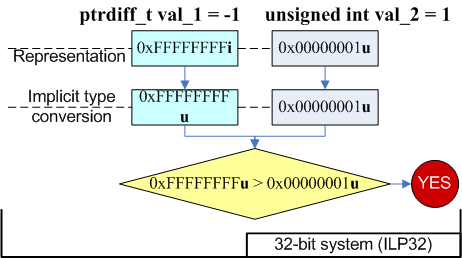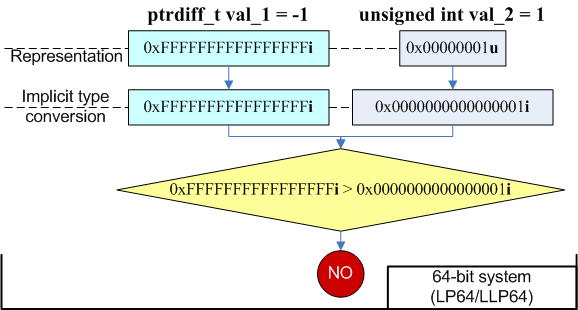﻿ Lesson 17. Pattern 9. Mixed arithmetic# Lesson 17. Pattern 9. Mixed arithmetic

I hope you have already rested from the 13-th lesson and now are ready to study one more important error pattern related to arithmetic expressions in which types of different capacities participate.

Mixed use of memsize-types and non-memsize types in expressions may lead to incorrect results on 64-bit systems and concern changes of the range of the input values. Consider some examples:

``````size_t Count = BigValue;
for (unsigned Index = 0; Index != Count; ++Index)
{ ... }``````

This is an example of an eternal loop occurring if Count > UINT_MAX. Suppose that this code works well on a 32-bit system with fewer iterations than UINT_MAX. But the 64-bit version of the program can process more data and may need more iterations. Since the values of Index variable lie within the range [0..UINT_MAX], the condition "Index != Count" will never be fulfilled and it leads to the eternal loop.

Note. Consider that this sample may work well at some particular settings of the compiler. Sometimes it is a source of much confusion because the code seems to be correct. In one of the following lessons we will tell you about phantom errors that reveal themselves only some time later. If you are already longing to learn why the code behaves so strangely, see the article "A 64-bit horse that can count".

To correct the code you should use only memsize-types in the expressions. In our example we may change the type of the variable Index from "unsigned" to size_t.

Another frequent error is using expressions of the following kind:

``````int x, y, z;
ptrdiff_t SizeValue = x * y * z;``````

We have already examined such examples with an arithmetic overflow that occurs when calculating expressions using non-memsize types. The result was incorrect of course. The search and detection of this code fragment was complicated by the fact that compilers usually do not generate any warnings on it. From the viewpoint of C++ language it is an absolutely correct construct: several variables of "int" type are multiplied together, after that the result is implicitly extended to the type ptrdiff_t and is assigned to a variable.

Here is a small code sample that shows the danger of inaccurate expressions with mixed types (these results were obtained in Microsoft Visual C++ 2005 in the 64-bit compilation mode):

``````int x = 100000;
int y = 100000;
int z = 100000;
ptrdiff_t size = 1;                   // Result:
ptrdiff_t v1 = x * y * z;             // -1530494976
ptrdiff_t v2 = ptrdiff_t (x) * y * z; // 1000000000000000
ptrdiff_t v3 = x * y * ptrdiff_t (z); // 141006540800000
ptrdiff_t v4 = size * x * y * z;      // 1000000000000000
ptrdiff_t v5 = x * y * z * size;      // -1530494976
ptrdiff_t v6 = size * (x * y * z);    // -1530494976
ptrdiff_t v7 = size * (x * y) * z;    // 141006540800000
ptrdiff_t v8 = ((size * x) * y) * z;  // 1000000000000000
ptrdiff_t v9 = size * (x * (y * z));  // -1530494976``````

All the operands in such expressions must be cast to a type of a larger capacity while performing the calculations. Remember that an expression like

``ptrdiff_t v2 = ptrdiff_t (x) + y * z;``

does not guarantee a correct result at all. It guarantees only that the expression "ptrdiff_t (x) + y * z" will have the type "ptrdiff_t".

So, if the expression's result must have a memsize-type, there must be only memsize-types in the expression too. Here is the correct version:

``ptrdiff_t v2 = ptrdiff_t (x) + ptrdiff_t (y) * ptrdiff_t (z); // OK!``

However, it is not always necessary to convert all the arguments to a memsize-type. If an expression consists of identical operators, you may convert only the first argument to the memsize-type. Consider an example:

``````int c();
int d();
int a, b;
ptrdiff_t v2 = ptrdiff_t (a) * b * c() * d();``````

The order of calculating the expression with the operators of the same priority has not been defined. More exactly, the compiler may choose any order of calculating the subexpressions (for example the calls of the functions c() and d()) it considers the most efficient, even if the subexpressions may cause side effects. The order of appearance of side effects has not been defined either. But since the multiplication operation refers to left-associative operators, the procedure of calculation will be performed in the following way:

``ptrdiff_t v2 = ((ptrdiff_t (a) * b) * c()) * d();``

As a result, each of the operators will be cast to the type "ptrdiff_t" before the multiplication and we will get the correct result.

Note. If there are integer calculations in your program and they need the control over overflows, resort to the class SafeInt - you may learn about its implementation and see its description in MSDN.

Mixed use of types may also result in the changes in program logic:

``````ptrdiff_t val_1 = -1;
unsigned int val_2 = 1;
if (val_1 > val_2)
printf ("val_1 is greater than val_2\n");
else
printf ("val_1 is not greater than val_2\n");
//Output on 32-bit system: "val_1 is greater than val_2"
//Output on 64-bit system: "val_1 is not greater than val_2"``````

According to C++ rules, the variable val_1 is extended to the type "unsigned int" and becomes the value 0xFFFFFFFFu on a 32-bit system - the condition "0xFFFFFFFFu > 1" is fulfilled. On a 64-bit system, however, it is the variable val_2 that gets extended to the type "ptrdiff_t" - in this case it is the expression "-1 > 1" which is checked. Figures 1 and 2 give the outlines of the transformations that take place.Figure 1 - Transformations taking place in the 32-bit version of the codeFigure 2 - Transformations taking place in the 64-bit version of the code

If you need to make the code behave in the same way as before, you should change the type of the variable val_2:

``````ptrdiff_t val_1 = -1;
size_t val_2 = 1;
if (val_1 > val_2)
printf ("val_1 is greater than val_2\n");
else
printf ("val_1 is not greater than val_2\n");``````

Actually, it would be more correct not to compare signed and unsigned types at all, but this issue lies beyond the current topic.

We have considered only simple expressions. But the described issues may occur when using other C++ constructs too:

``````extern int Width, Height, Depth;
size_t GetIndex(int x, int y, int z) {
return x + y * Width + z * Width * Height;
}
...
MyArray[GetIndex(x, y, z)] = 0.0f;``````

If there is a large array (containing more than INT_MAX items), this code will be incorrect and we will be directed to the wrong items of the array MyArray. Although it is the value of "size_t" type which is returned, the expression "x + y * Width + z * Width * Height" is calculated using the type "int". I think you have already guessed what the corrected code will look like:

``````extern int Width, Height, Depth;
size_t GetIndex(int x, int y, int z) {
return (size_t)(x) +
(size_t)(y) * (size_t)(Width) +
(size_t)(z) * (size_t)(Width) * (size_t)(Height);
}``````

Or a bit simpler:

``````extern int Width, Height, Depth;
size_t GetIndex(int x, int y, int z) {
return (size_t)(x) +
(size_t)(y) * Width +
(size_t)(z) * Widt) * Height;
}``````

In the next example again we have a mixture of a memsize-type (the pointer) and a 32-bit "unsigned" type:

``````extern char *begin, *end;
unsigned GetSize() {
return end - begin;
}``````

The result of the expression "end - begin" has the type "ptrdiff_t". Since the function returns the type "unsigned", there occurs an implicit type conversion that leads to a loss of the more significant bits of the result. So, if the pointers begin and end refer to the beginning and the end of the array whose size is more than UINT_MAX (4Gb), the function will return an incorrect result.

And one more example. Here we are going to consider not a returned value but a formal argument of a function:

``````void foo(ptrdiff_t delta);
int i = -2;
unsigned k = 1;
foo(i + k);``````

This code resembles an example with incorrect pointer arithmetic discussed in the 13-th lesson, does not it? Right, here we have the same. We get the incorrect result when the actual argument, equaling 0xFFFFFFFF and having the type "unsigned", is implicitly extended to the type "ptrdiff_t".

## Diagnosis

Errors occurring in 64-bit systems when integer types and memsize-types are used together are presented in many C++ syntactic constructs. To diagnose these errors several diagnostic warnings are used. PVS-Studio analyzer warns the programmer about possible errors with the help of these warnings: V101, V103, V104, V105, V106, V107, V109, V110, V121.

``````int c();
int d();
int a, b;
ptrdiff_t x = ptrdiff_t(a) * b * c() * d();``````

Although the expression itself multiplies together the arguments extending their types to "ptrdiff_t", an error may hide in the procedure of calculating these arguments. That is why the analyzer still warns you about the mixed types: "V104: Implicit type conversion to memsize type in an arithmetic expression".

PVS-Studio tool also allows you to find potentially unsafe expressions which hide behind explicit type conversions. To enable this function you should enable the warnings V201 and V202. By default, the analyzer does not generate warnings concerning explicit type conversions. For example:

``````TCHAR *begin, *end;
unsigned size = static_cast<unsigned>(end - begin);``````

The warnings V201 and V202 will help you detect such incorrect code fragments.

Still the analyzer will pay no attention to type conversions which are safe from the viewpoint of the 64-bit code:

``````const int *constPtr;
int *ptr = const_cast<int>(constPtr);
float f = float(constPtr);
char ch = static_cast<char>(sizeof(double));``````

The course authors: Andrey Karpov (karpov@viva64.com), Evgeniy Ryzhkov (evg@viva64.com).

The rightholder of the course "Lessons on development of 64-bit C/C++ applications" is OOO "Program Verification Systems". The company develops software in the sphere of source program code analysis. The company's site: http://www.viva64.com.##### Use PVS-Studio to search for bugs in C, C++, C# and Java

We offer you to check your project code with PVS-Studio. Just one bug found in the project will show you the benefits of the static code analysis methodology better than a dozen of the articles.

410
14 111

### Do you make errors in the code?